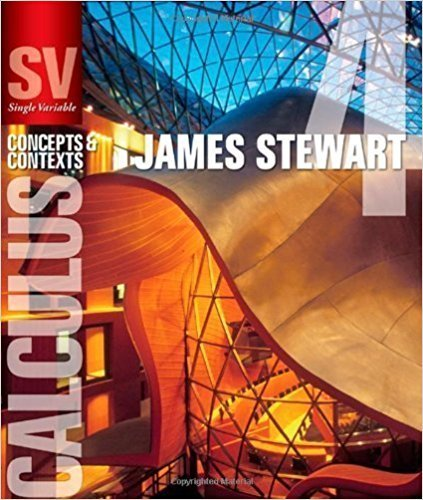×
×

# Solutions for Chapter 7.3: SEPARABLE EQUATIONS## Full solutions for Single Variable Calculus: Concepts and Contexts (Stewart's Calculus Series) | 4th Edition

ISBN: 9780495559726Solutions for Chapter 7.3: SEPARABLE EQUATIONS

Solutions for Chapter 7.3
4 5 0 358 Reviews
21
3
##### ISBN: 9780495559726

This textbook survival guide was created for the textbook: Single Variable Calculus: Concepts and Contexts (Stewart's Calculus Series), edition: 4. This expansive textbook survival guide covers the following chapters and their solutions. Chapter 7.3: SEPARABLE EQUATIONS includes 54 full step-by-step solutions. Single Variable Calculus: Concepts and Contexts (Stewart's Calculus Series) was written by and is associated to the ISBN: 9780495559726. Since 54 problems in chapter 7.3: SEPARABLE EQUATIONS have been answered, more than 20091 students have viewed full step-by-step solutions from this chapter.

Key Calculus Terms and definitions covered in this textbook
• Completing the square

A method of adding a constant to an expression in order to form a perfect square

• Confounding variable

A third variable that affects either of two variables being studied, making inferences about causation unreliable

• Ellipse

The set of all points in the plane such that the sum of the distances from a pair of fixed points (the foci) is a constant

• Equivalent vectors

Vectors with the same magnitude and direction.

• Interquartile range

The difference between the third quartile and the first quartile.

• Linear factorization theorem

A polynomial ƒ(x) of degree n > 0 has the factorization ƒ(x) = a(x1 - z1) 1x - i z 22 Á 1x - z n where the z1 are the zeros of ƒ

• Logarithmic function with base b

The inverse of the exponential function y = bx, denoted by y = logb x

• Magnitude of a real number

See Absolute value of a real number

• nth power of a

The number with n factors of a , where n is the exponent and a is the base.

• Number line graph of a linear inequality

The graph of the solutions of a linear inequality (in x) on a number line

• Obtuse triangle

A triangle in which one angle is greater than 90°.

• Open interval

An interval that does not include its endpoints.

A function that can be written in the form ƒ(x) = ax 2 + bx + c, where a, b, and c are real numbers, and a ? 0.

• Quartile

The first quartile is the median of the lower half of a set of data, the second quartile is the median, and the third quartile is the median of the upper half of the data.

• Rational numbers

Numbers that can be written as a/b, where a and b are integers, and b ? 0.

• Sequence

See Finite sequence, Infinite sequence.

• Slant line

A line that is neither horizontal nor vertical

• Transpose of a matrix

The matrix AT obtained by interchanging the rows and columns of A.

• Trichotomy property

For real numbers a and b, exactly one of the following is true: a < b, a = b , or a > b.

• Xmin

The x-value of the left side of the viewing window,.

×